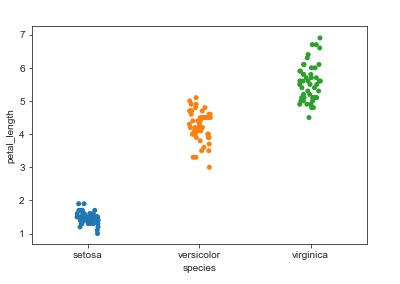# How to avoid the points getting overlapped while using stripplot in categorical scatter plot Seaborn Library in Python?

Visualizing data is an important step since it helps understand what is going on in the data without actually looking at the numbers and performing complicated computations. Seaborn is a library that helps in visualizing data. It comes with customized themes and a high level interface.

General scatter plots, histograms, etc can’t be used when the variables that need to be worked with are categorical in nature. This is when categorical scatterplots need to be used.

Plots such as ‘stripplot’, ‘swarmplot’ are used to work with categorical variables. The ‘stripplot’ function is used when atleast one of the variables is categorical. The data is represented in a sorted manner along one of the axes. But the disadvantage is that certain points get overlapped. This where the ‘jitter’ parameter has to be used to avoid the overlapping between variables.

It adds some random noise to the dataset, and adjusts the positions of the values along the categorical axis.

Syntax of stripplot function

seaborn.stripplot(x, y,data, jitter = …)

Let us see how ‘jitter’ parameter can be used to plot categorical variables in a dataset −

## Example

import pandas as pd
import seaborn as sb
from matplotlib import pyplot as plt
sb.stripplot(x = "species", y = "petal_length", data = my_df, jitter = True)
plt.show()

## Output## Explanation

• The required packages are imported.
• The input data is ‘iris_data’ which is loaded from the scikit learn library.
• This data is stored in a dataframe.